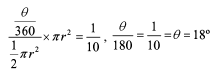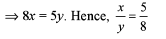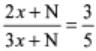Courses

Important Questions Test: Ratio & Proportion- 2

15 Questions MCQ Test UPSC Prelims Paper 2 CSAT - Quant, Verbal & Decision Making | Important Questions Test: Ratio & Proportion- 2

Description
Attempt Important Questions Test: Ratio & Proportion- 2 | 15 questions in 30 minutes | Mock test for Quant preparation | Free important questions MCQ to study UPSC Prelims Paper 2 CSAT - Quant, Verbal & Decision Making for Quant Exam | Download free PDF with solutions
QUESTION: 1

What is the angle in degrees made by a sector, the ratio of whose area with the area of the semicircle is equal to 1:10?

Solution:

Area of semicircle = (1/2)πr2
Area of sector = (θ/360)πr2QUESTION: 2

The value of a diamond varies directly as the square of its weight. If a diamond worth Rs 10,000 is divided into 2 pieces in the ratio of 4:6, what is the loss in value?

Solution:

V ∝ W2, V = KW2
Where, V = Value of diamond, W = weight of diamond and K = constant
⇒ 10000 = K.(10)2
⇒ K = 100
Hence, V = 100 x 42 = 1600
also, V = 100 x 62 = 3600
Net loss = Rs.(10000 - 5200) = Rs 4800 i.e., 48%

QUESTION: 3

The first, second and third class fares between two railway stations, Patna and Lucknow were 10:8:3 and the number of first, second and third class passengers between the two stations was is 3:4:10. If total sales of the ticket is Rs 16,100, find the money obtained by the sales of second class tickets.

Solution:

► Let the fare of 1st, 2nd and 3rd class be 10x, 8x, and 3x respectively.
► Let the no. of passengers of 1st, 2nd and 3rd class be 3y, 4y and 10y respectively.
Then 30xy + 32xy + 30xy = 16100
⇒ 92xy = 16100
Hence xy = 175
Required amount = 32xy = 32 x 175 = 5600

QUESTION: 4

Two horses cost as much as 5 dogs, 6 dogs as much as 8 oxen, 10 oxen as much as 50 sheep, 14 sheep as much as 9 goats. If the price of one goat is Rs 70, how much will one horse cost?

Solution:

► Price of one goat = Rs 70 ⇒ Price of 9 goats = 9 x 70 = Rs 630
► Price of 14 sheeps = Rs 630
⇒ Price of 1 sheep = Rs 630/14 = Rs 45 ⇒ Price of 50 sheep = Rs 45 x 50 = Rs 2250
► Price of 10 oxen = 2250 ⇒ Price of 1 oxen = 225 ⇒ Price of 8 oxen  = 1800
► Price of 6 dogs = 1800 ⇒ Price of 1 dog = 300 ⇒ Price of 5 dog = 1500
► Price of two horses = 1500 ⇒ Price of one horse = 750

QUESTION: 5

A is proportional to B. B is inversely proportional to C. C is proportional to the square of D. D is directly proportional to the cube root of E. Assuming positive integers, if A increases then E

Solution:

Given,
B
B ∝ (1/C)
C ∝ D2
∛E
When A increases ⇒ B also increases ⇒ C decreases
Decrease in C results in decrease in D and decrease in D results decrease in E.

QUESTION: 6

The sum of four numbers is 253. The ratio of the first number to the second number is 2:3. The ratio of the second number to the third number is 5:6. The ratio of the third number to the fourth number is 8:9. What is the average of the second number and the third number?

Solution:

► Let the 1stno. = A = x
2nd no.= B , 3rd no.= C , 4th no. = D
► A:B = 2:3
A/B = 2/3
x/B = 2/3
B = 3x/2
► B:C = 5:6
(3x/2)/C = 5/6
C = (6*3x)/2*5 = 9x/5
C = 9x/5
► C:D = 8:9
9x/5/D = 8/9
D = (9x*9)/8*5 = 81x/40
D = 81x/40
► A + B + C + D = 253. (Given)
⇒ x + 3x/2 + 9x/5 + 81x/40 = 253
⇒ (40x+ 60x+ 72x+81x)/40 = 253
⇒ 253x = 253*40
⇒ X = (253*40)/253 = 40
⇒ 1st no.(A) = X = 40
⇒ 2nd no.(B) = 3x/2 =( 3 × 40)/2 = 60
⇒ 3rd no.(C) = 9x/5 = (9×40)/5 = 72
⇒ 4th no.(D) = 81x/40 =( 81×40/)/40 = 81
► Average of numbers = sum of observations/ total no.of observations
► Average of 2nd no. & 3rd no.= (60+72)/2 = 132/2 = 66

QUESTION: 7

A man has rs.480 in the denominations of one-rupee notes, five-rupee notes and ten-rupee notes. The number of notes of each denomination is equal. What is the total number of notes that he has ?

Solution:

► Given that a man has Rs. 480 in the denominations of one-rupee notes, five-rupee notes and ten-rupee notes. The number of notes of each denomination is equal.
► We are to find the total number of notes he has.
► Let x represents the number of notes of each of the three denominations.
► Then, according to the given information, we have
⇒ 1.x + 5.x + 10.x = 480
⇒ x + 5x + 10x = 480
⇒ 16x = 480
⇒ x = 30
► Therefore, the total number of notes is given by
⇒ x + x + x = 3x = 3*30 = 90

QUESTION: 8

Three friends A, B and C started a venture with capitals in the ratio of 4:1:15. At the end of every quarter A halves his capital, while B doubles his capital and C leaves his capital untouched. This process is repeated till the end of the year. If at the end of the year B ’ s share of the profit is Rs 22,000, what is the total profit?

Solution:

► Let A, B, C invested Rs 4x, Rs x, Rs 15x respectively.
► According to question, Ratio of their investments for 1 year
= (4x*3 + 2x*3 + x*3 + x/2*3) : (x*3 + 2x*3 + 4x*3 + 8x*3) : (15x*12)
⇒ 45x/2 : 45x : 180x
⇒ 45 : 90 : 360
⇒ 1 : 2 : 8
Total profit = (11/2)*22000 = Rs 121000

QUESTION: 9

Gandhiji owns cows, some black and some white. He finds that 4 black cows and 3 white cows gave the same amount of milk in 5 days as 3 black cows and 5 white cows gave in 4 days. What is the ratio of milk given by a black cow in a day to that given by a white cow in a day?

Solution:

► Let 1 black cow gives x litres milk in 1 day and 1 white cow gives y litres milk in 1 day.
► Then 5(4x + 3y) = 4(3x + 5y)
⇒ 20x + 15y = 12x + 20yQUESTION: 10

A metal trader buys 2 kinds of silver foils, the ratio of their prices being 1:4. He sells the alloy at Rs 90 per kg so that he can make a profit of 20%. If the ratio of their quantities present in a alloy is 6:1 respectively, find the purchase price of the foil present in lesser quantity.

Solution:
QUESTION: 11

Read the passage below and solve the questions based on it.
A book having pages between 4,000 and 5,000 is divided into four pans, each part being divided into chapters. The total number of pages in each of the four parts is the same. The ratio of the chapters across all the parts is 6:5:10:14. The number of chapters in the fourth part is 70

Q.

What is the total number of pages in the book?

Solution:

► According to question, A:B:C:D = 6:5:10:14
► Now in D there are 70 chapters
Therefore, A:B:C:D = 30:25:50:70      [ Multiplying Each by 5 ]
► Total Chapters = 30 + 25 + 50 + 70 = 175
L.C.M of 30, 25, 50, 70 is 1050
So each part should have 1050 pages.
So total pages = 4 x 1050 = 4200 ans.

QUESTION: 12

The ratio of the age of Sita and her mother is 2:3. N years from now, ratio of their ages will become 3:5. What is the value of N?

Solution:

► Let their present ages be 2x and 3x.
Given that10x + 5N = 9x + 3N
X = -2N which 18 not possible.
Hence, correct option is (d).

QUESTION: 13

P works twice as fast as Q, whereas P and Q together can work three times as fast as R. If P, Q and R together work on a job, in what ratio should they share the earnings?

Solution:

► If P is taking 3 days to do some work, then Q takes 6 days to do the same work.
► Now, both of them will take 2 days to complete the work. So, R takes 6 days to complete the same work.
► Hence, earning should be distributed in the ratio of their efficiency, i.e., 2 : 1 : 1.

QUESTION: 14

Read the passage below and solve the questions based on it.
There are certain number of apples, guavas and oranges in a basket. The number of each variety is more than one. The ratio of the number of apples to the number of guavas is equal to the ratio of the number of guavas to the number of oranges.

Q.

If the total number of fruits is 61, then find the number of guavas.

Solution:

► Number of apples/Number of guavas = Number of guavas/Number of oranges
► (Number of guavas)2 = Number of apples x Number of oranges.
► This gives us a hint that Number of apples x Number of oranges should be a prefect square.
Now start using the options.
Option (b) satisfies the relationship (16/20 = 20/25)

QUESTION: 15

Anand and Bhavana have a few one rupee, 50 paise and 25 paise coins each. The total amount with each of them is Rs 5. The number of coins of two of the three types of coins that Anand has are equal and same is the case for the coins that Bhavana has. If Anand has the maximum possible total number of coins, then find the maximum possible total number of coins that Bhavana can have given that she has fewer coins than Anand.

Solution:

2(x+0.5x+0.25)=104x

= 10X = 2.5
0.5x=0.5*2.5

=12.5~12Use Code STAYHOME200 and get INR 200 additional OFF Use Coupon Code

Track your progress, build streaks, highlight & save important lessons and more!

Similar ContentRelated tests